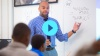# Systems of Linear Equations

Students explore what happens when you consider two linear equations simultaneously, and explore the many rich applications that can be modeled with systems of linear equations in two variables.

Math

Unit 6

## Unit Summary

In Unit 6, eighth-grade students explore what happens when you consider two linear equations simultaneously. They graph two lines in the same coordinate plane and ask themselves what coordinate points satisfy both of the equations. They consider what it means when two lines never intersect or when they overlap completely. Students learn algebraic methods that can be used to solve systems when graphing is not efficient. Using the structure of the equations in a system, students will determine if systems have one, no, or infinite solutions without solving the system (MP.7). Students also explore the many rich applications that can be modeled with systems of linear equations in two variables (MP.4).

Students will use their knowledge from previous eighth-grade units, including work with single linear equations and functions from clusters 8.EE.B and 8.F.B. They will also need to draw on concepts from sixth grade, where they understood solving an equation as a process of answering which values make an equation true.

In high school, students will continue their work with systems, working with linear, absolute value, quadratic, and exponential functions. They will also graph linear inequalities and consider what the solution of a system of linear inequalities looks like in the coordinate plane.

Pacing: 15 instructional days (11 lessons, 3 flex days, 1 assessment day)

Fishtank Plus for Math

Unlock features to optimize your prep time, plan engaging lessons, and monitor student progress.## Assessment

The following assessments accompany Unit 6.

### Pre-Unit

Have students complete the Pre-Unit Assessment and Pre-Unit Student Self-Assessment before starting the unit. Use the Pre-Unit Assessment Analysis Guide to identify gaps in foundational understanding and map out a plan for learning acceleration throughout the unit.

### Mid-Unit

Have students complete the Mid-Unit Assessment after lesson 6.

### Post-Unit

Use the resources below to assess student mastery of the unit content and action plan for future units.

Expanded Assessment Package

Use student data to drive your planning with an expanded suite of unit assessments to help gauge students’ facility with foundational skills and concepts, as well as their progress with unit content.

## Unit Prep

### Intellectual Prep

Unit Launch

Prepare to teach this unit by immersing yourself in the standards, big ideas, and connections to prior and future content. Unit Launches include a series of short videos, targeted readings, and opportunities for action planning.#### Internalization of Standards via the Post-Unit Assessment

• Take the Post-Unit Assessment. Annotate for:
• Standards that each question aligns to
• Strategies and representations used in daily lessons
• Relationship to Essential Understandings of unit
• Lesson(s) that Assessment points to

#### Internalization of Trajectory of Unit

• Read and annotate the Unit Summary.
• Notice the progression of concepts through the unit using the Lesson Map.
• Essential Understandings
• Connection to Post-Unit Assessment questions
• Identify key opportunities to engage students in academic discourse. Read through our Teacher Tool on Academic Discourse and refer back to it throughout the unit.

### Essential Understandings

• A system of linear equations is a set of two or more linear equations that involve the same, related variables.
• The solution to a system of linear equations represents all of the points that satisfy all of the equations in the system simultaneously. This is seen graphically as the intersecting or overlapping points on the graph and can be verified algebraically by confirming the coordinate point(s) satisfy the equations when they are substituted in.
• Systems of equations can have one unique solution (intersecting lines), no solution (non-overlapping parallel lines), or infinite solutions (completely overlapping lines).
• Systems of equations are useful to model real-world situations when more than one equation and more than one variable are involved.

### Vocabulary

elimination (linear combinations)

infinite solutions

no solution

substitution (to solve a system of equations)

system of linear equations

unique solution

zero pair

To see all the vocabulary for Unit 6, view our 8th Grade Vocabulary Glossary.

## Lesson Map

Topic A: Analyze & Solve Systems of Equations Graphically

Topic B: Analyze & Solve Systems of Equations Algebraically

## Common Core Standards

Key

Major Cluster

Supporting Cluster

### Core Standards

#### Expressions and Equations

• 8.EE.C.8 — Analyze and solve pairs of simultaneous linear equations.
• 8.EE.C.8.A — Understand that solutions to a system of two linear equations in two variables correspond to points of intersection of their graphs, because points of intersection satisfy both equations simultaneously.
• 8.EE.C.8.B — Solve systems of two linear equations in two variables algebraically, and estimate solutions by graphing the equations. Solve simple cases by inspection. For example, 3x + 2y = 5 and 3x + 2y = 6 have no solution because 3x + 2y cannot simultaneously be 5 and 6.
• 8.EE.C.8.C — Solve real-world and mathematical problems leading to two linear equations in two variables. For example, given coordinates for two pairs of points, determine whether the line through the first pair of points intersects the line through the second pair.

• 6.EE.B.5
• 8.EE.B.6
• 8.EE.C.7

• 8.F.B.4

• A.CED.A.3

• A.REI.C.5
• A.REI.C.6
• A.REI.C.7
• A.REI.C.8
• A.REI.C.9

### Standards for Mathematical Practice

• CCSS.MATH.PRACTICE.MP1 — Make sense of problems and persevere in solving them.

• CCSS.MATH.PRACTICE.MP2 — Reason abstractly and quantitatively.

• CCSS.MATH.PRACTICE.MP3 — Construct viable arguments and critique the reasoning of others.

• CCSS.MATH.PRACTICE.MP4 — Model with mathematics.

• CCSS.MATH.PRACTICE.MP5 — Use appropriate tools strategically.

• CCSS.MATH.PRACTICE.MP6 — Attend to precision.

• CCSS.MATH.PRACTICE.MP7 — Look for and make use of structure.

• CCSS.MATH.PRACTICE.MP8 — Look for and express regularity in repeated reasoning.

Unit 5

Linear Relationships

Unit 7

Pythagorean Theorem and Volume